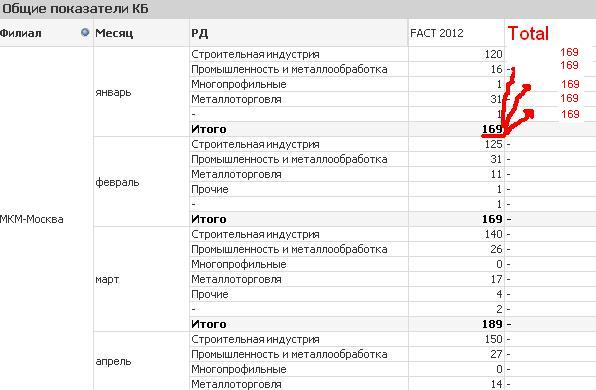# New to QlikView

Discussion board where members can get started with QlikView.

HighlightedNot applicable

## total field

Hi !

I have table with expression (FACT 2012):

sum(if((Aggr(sum({<year={"2012"}, bg_t={'*'}, bg_t-={'b\g}, Holding=>} Quantity),AffiliateID, Holding,mes))>0 ,1,0))

How can i get field with total sum?Tags (3)
1 Solution

Accepted SolutionsMVP & Luminary

## Re: total field

Try adding total <Dim1,Dim2> to the sum expression. Replace Dim1 and Dim2 with the first two dimensions of your pivot table.

sum(total <Dim1,Dim2>  if((Aggr(sum({<year={"2012"}, bg_t={'*'}, bg_t-={'b\g}, Holding=>} Quantity),AffiliateID, Holding,mes))>0 ,1,0))

See attached example for how it works.

talk is cheap, supply exceeds demand
7 RepliesNot applicable

## Re: total fieldMVP & Luminary

## Re: total field

Try adding total <Dim1,Dim2> to the sum expression. Replace Dim1 and Dim2 with the first two dimensions of your pivot table.

sum(total <Dim1,Dim2>  if((Aggr(sum({<year={"2012"}, bg_t={'*'}, bg_t-={'b\g}, Holding=>} Quantity),AffiliateID, Holding,mes))>0 ,1,0))

See attached example for how it works.

talk is cheap, supply exceeds demandNot applicable

## Re: total field

Thank you, Gysbert for your help!

I put exemple in you attachment, please lookMVP & Luminary

## Re: total field

Hi,

Try this expression

sum(Total<Year, Product, Region>if((Aggr(sum({<year={"2008"}, Product={'*'}, product-={'Banana'}, Holding=>} Sales),Year, Product,Month))>0 ,1

,0))

Regards,

jagan.Not applicable

## Re: total field

thank you very much!Not applicable

## Re: total field

thank you, it worksEmployee

## Re: total field

I'm wondering whether this approach can work for total <vMyVariable>?

It works fine: if within <> I write MyField , the formula then looks the following way:  sum(VALUE) / sum(total <MyField>  VALUE)

PROBLEM: what if MyField variates due to the drill down, so that it can be MyField1,MyField2,MyField3?

It does not work: if within <> I write vMyVariable containing the needed field, the formula then looks the following way:  sum(VALUE) / sum(total <vMyVariable>  VALUE)

Any ideas/suggestions are very much appreciated.